# I think my problem solving is absurd.

## Homework Statement

Evaluate the definite integral: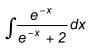## Homework Equations

$$\int u^n du/dx$$ = u^(n+1)/(n+1) (n not equal to -1)

## The Attempt at a Solution

(-1) muliplied by $$\int$$ (e^-x +2)^-1 (-e^-x) dx = ?

and if I follow the equation above, I got denominator zero, for I broke the rules 'n is not equal to zero'
I think I did as the equation. but now what should I do now?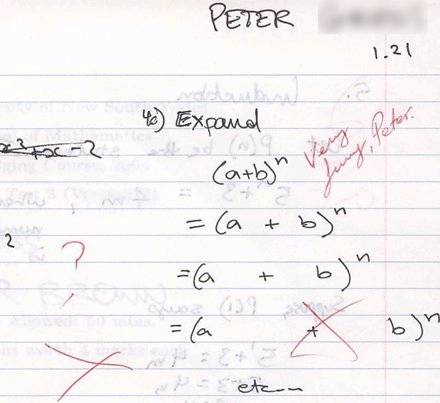Homework Helper
Do you know how to integrate

$$\int \frac 1 u \, du$$
?

Let u = e^(-x) + 2
the du = ...

-e^(-x)

Homework Helper
So with $$u = e^{-x} + 2$$ and $$du = -e^{-x}$$, what happens to your integral?

So with LaTeX Code: u = e^{-x} + 2 and LaTeX Code: du = -e^{-x} , what happens to your integral?

Thank you for your help. I did like this: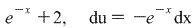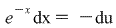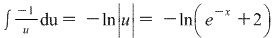Can you check is this the right answer?

Looks good. You are correct that the absolute value signs are not needed in the answer, since $$e^{-x} + 2$$ is never negative.